# How to create code questions

Quarterfall has special functionality for Computer Science and ICT education. In particular, you can easily create questions in which student must provide some code in a programming language. Quarterfall helps them enter this code, and can run unit tests to check the answers of the students.

### Adding programming code in questions

When teaching about programming, you often want to provide code snippets in your assignment texts. This is very easy. Wherever you can provide text (like in study material, question texts, answers, solutions, or feedback) you can add code in various programming languages. This code is then displayed to the students with syntax highlighting and line numbers.

To add a piece of code, start a line with three backquotes, followed by the name of the programming language. Next, type the code, and end with a line with three back quotes. Here is an example: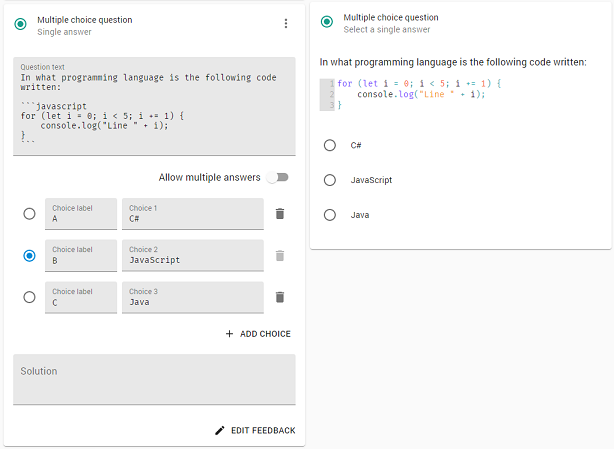### Creating a code question

The more interesting option is to add code questions to assignments. To this end, create a new course, add a module, and add an assignment, called "Power", as we are going to create an assignment in which students must compute the power of a number. Press Add block and select the Code question.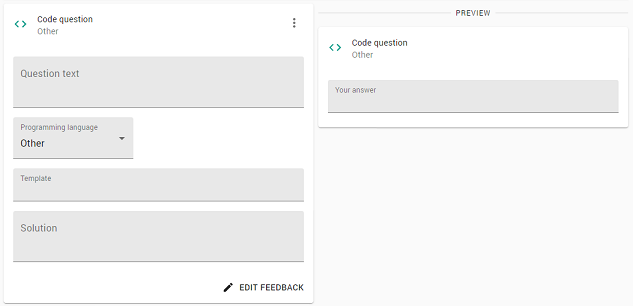The first thing we are going to do is to set the programming language that must be used by the student. This has two effects. First of all, it specialized the editor that the students can use to provide their answers, such that it does the correct syntax highlighting. And secondly, it defines how unit tests are performed when we want to automatically check the answers of the students.

In this tutorial, we will use C# as a language, but Quarterfall supports many other languages, and we will increase the number all the time. Press the Programming language drop-down menu and select C#. (Note that the language is indicated in the preview.)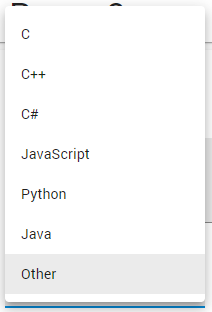Now provide a question text and, maybe, a solution. So the assignment will look as follows, where we already filled in a possible answer by a student.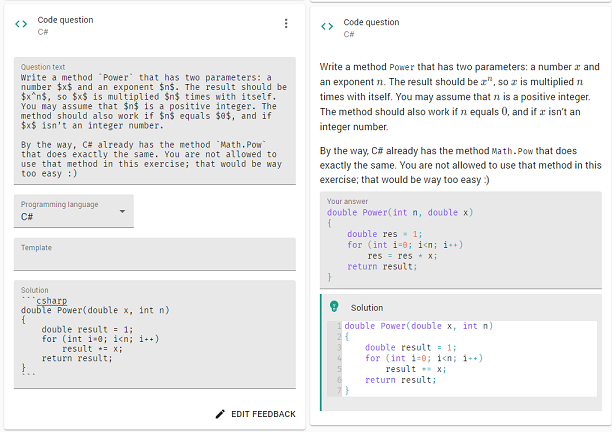Note that in the solution we used the construction to add programming code, as indicated above. The solution is obviously not shown to the student when making the exercise. Only when the student completes the question (and can no longer change it) is the solution shown.

### Templates

As the example above shows, the student might not provide the answer in the way you intended (He reversed the arguments). This can lead to confusion when you provide a solution. And it is killing when you want to automatically run unit tests.

To remedy this, you can provide a template for the answer of the student. The template you provide is automatically filled in, and the student can then extend it to complete the answer. For example, you could provide the following template: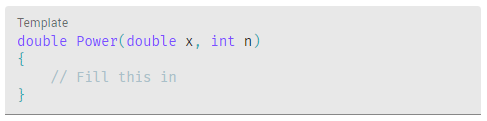Let us first do some simple test to see whether the student uses the correct language constructs. In particular, we want to check whether the student uses a loop and does not use the Math.Pow method.

To this end, press Edit feedback below the code exercise. Next, use the Add Action button and add a feedback action. (For more information about adding feedback, check the tutorial on assignments.) As condition use answer.includes("Math.Pow"), and as feedback text give something like "You are not allowed to use the Math.Pow method."

For the loop check, add a second feedback action with conditions !includes(answer, "for") && !includes(answer, "while") and provide some relevant feedback text. So the actions will look as follows: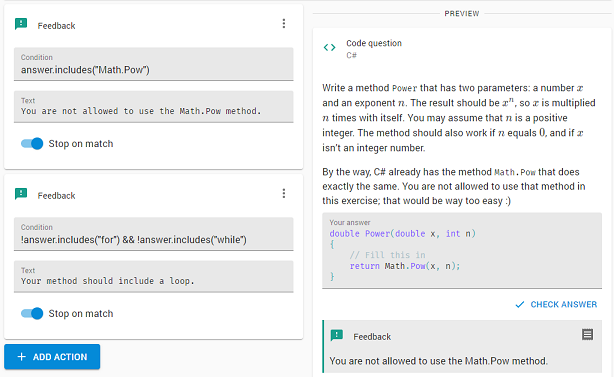Note that we switched on Stop on match such that no further feedback actions are executed anymore.

You can use this approach to test for many aspects of the answer. If you need more power, you can use a code action instead.

### Unit tests

Although feedback actions can check various aspects of the answer, they cannot test whether the code is correct and computes the right result. For this, Quarterfall makes it easy to perform unit tests on the code. When using unit tests, the answer of the student is compiled (if the language requires this) and then the method (or function) is called and the result is compared with the correct result.

To add a unit test, again click on Add action and select Unit test. The following, empty, unit test block appears: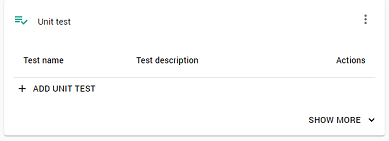Press the button Add unit test to add a test. A form appears where you can give the test a name, provide an optional description, and an expression that must be tested. This expression will normally contain a call to the method or function written by the student, so Power in our case.

Let us start with a simple test to see whether the case n equals 0 is handled correctly. As a test name give PowerZero and as condition Power(23,0) == 1 && Power(0,0) == 1. (Note that this condition must be in the programming language used. So for a different language this will look differently.)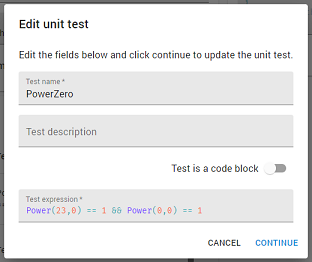To check it, type in a correct answer code in the preview and press the button Check Answer. The following should be shown: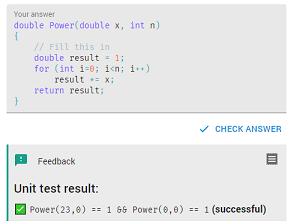As indicated, the unit test succeeded. If you made a mistake, it would have failed. If you would have provided a description for the test, the description will be shown, rather than the expression. You can add many more tests this way, to test all aspects of the answer.

As you will have noted, computing the result of the unit test will take some time. This is caused by the fact that the program must be compiled. This happens on our special unit test server. For languages that do not require compilation, like JavaScript and Python, this is a lot faster.

You might wonder what happens when you made a syntax error in the program. In this case an error occurs during the testing, which is reported to the student. Also, the student can see a log to find out what went wrong.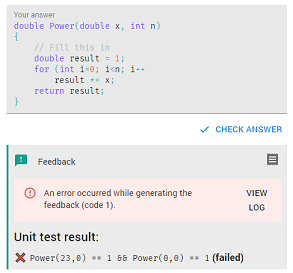### Multiple unit tests

The approach shown works fine if you you want to do just a few tests. If you want to do many different tests on the answer you can use a different approach. Press Add unit test to add another test to the action. This time enable the switch Test is a code block. Now you can type in any code you want to do tests. Note that this code must return a Boolean indicating whether the tests succeeded. Here is an example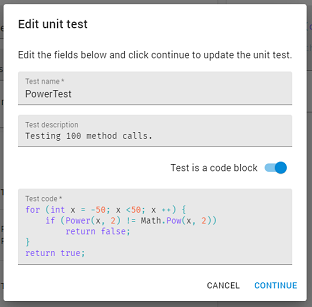You better test your unit tests carefully, because errors in your tests will obviously look like errors in the student answer.